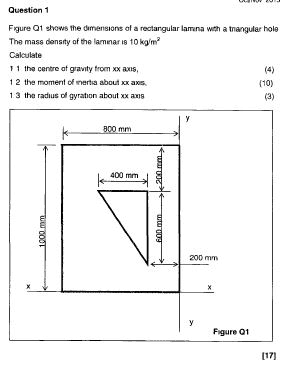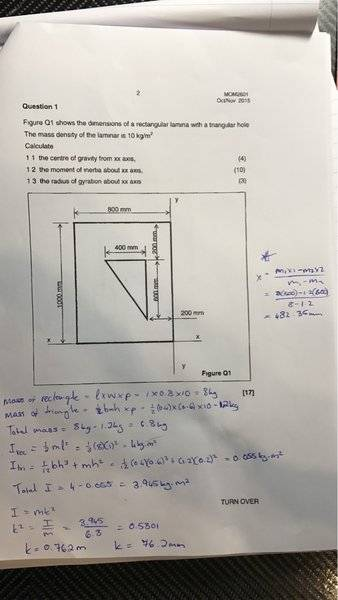# Centre of gravity, moment of inertia and radius of gyration

## Homework Statement## Homework Equations

Centre of gravity: X=m1x1-m2x2/m1-m2

MOI rectangle: 1/3ml^2

MOI triangle: 1/18md^2

## The Attempt at a Solution

Mass of body 1: b*l*p = 0.8*1*10=8kg

Mass of body 2: 1/2b*h*p = 1/2(0.4)*0.6*10=1.2kg

1.1

X=m1x1-m2x2/m1-m2

= 8*(-400)-1.2*(-1000/3)/8-1.2

= -411.7mm

1.2

Ixx= 1/3ml^2 - 1/18md^2

= 1/3*8*1^2 - 1/18*1.2*0.2^2

=2.664m^4

1.3

Ixx=mk^2

2.664=(8-1.2)*k^2

k=625.91mm

haruspex
Homework Helper
Gold Member
2020 Award
= 8*(-400)-1.2*(-1000/3)/8-1.2
How do you get 400, why -400, and how do you get 1000/3?

How do you get 400, why -400, and how do you get 1000/3?

That should be 500, from the centre of the rectangle to the XX axis is 500mm.
I believe the centre of the triangle should be 2/3*600 = 400mm
Then added to the 200mm from the start of the triangle to the XX axis should give 400+200=600mm.
I put a negative in front of the 400 and 1000/3 as they are "away" from the XX axis.
I don't know why I put 1000/3, I think I was dividing the length of the rectangle in 3 because of the triangle for some reason.

X=m1x1-m2x2/m1-m2

= 8*(-500)-1.2*(-600)/8-1.2

= -482.35mm

haruspex
Homework Helper
Gold Member
2020 Award
That should be 500, from the centre of the rectangle to the XX axis is 500mm.
I believe the centre of the triangle should be 2/3*600 = 400mm
Then added to the 200mm from the start of the triangle to the XX axis should give 400+200=600mm.
Right.
I put a negative in front of the 400 and 1000/3 as they are "away" from the XX axis.
But they are "away" from that axis in the positive y direction.

Right.

But they are "away" from that axis in the positive y direction.

Meaning they should be positive?
If they were below the X axis then they would be negative? In other words if the XX axis were at the top of the rectangle.

haruspex
Homework Helper
Gold Member
2020 Award
Meaning they should be positive?
If they were below the X axis then they would be negative? In other words if the XX axis were at the top of the rectangle.
Yes.

Okay so then

X=m1x1-m2x2/m1-m2

= 8*(500)-1.2*(600)/8-1.2

= 482.35mm

Is this correct?

haruspex
Homework Helper
Gold Member
2020 Award
Ixx= 1/3ml^2 - 1/18md^2
The term for the triangle is wrong. What axis would be right for that expression?

The term for the triangle is wrong. What axis would be right for that expression?

The formulae given for a rectangular triangle:

I=1/18md^2
I=1/18mh^2

because we want the inertia about xx axis I used =1/18md^2 where d=distance to xx axis=200mmharuspex